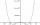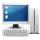# Three members GP

The sum of three numbers in GP (geometric progression) is 21 and the sum of their squares is 189. Find the numbers.

Result

a =  3
b =  6
c =  12
a2 =  12
b2 =  6
c2 =  3

#### Solution:Checkout calculation with our calculator of quadratic equations.Leave us a comment of example and its solution (i.e. if it is still somewhat unclear...):

Showing 0 comments:Be the first to comment!#### To solve this example are needed these knowledge from mathematics:

Looking for help with calculating roots of a quadratic equation? Do you have a linear equation or system of equations and looking for its solution? Or do you have quadratic equation?

## Next similar examples:

1. Theorem proveWe want to prove the sentence: If the natural number n is divisible by six, then n is divisible by three. From what assumption we started?
2. Five membersWrite first 5 members geometric sequence and determine whether it is increasing or decreasing: a1 = 3 q = -2
3. GP - 8 itemsDetermine the first eight members of a geometric progression if a9=512, q=2
4. Geometric sequence 4It is given geometric sequence a3 = 7 and a12 = 3. Calculate s23 (= sum of the first 23 members of the sequence).
5. Tenth memberCalculate the tenth member of geometric sequence when given: a1=1/2 and q=2
6. BiquadraticBy introducing a new variable solve biquadratic equation: ?
7. Six termsFind the first six terms of the sequence a1 = -3, an = 2 * an-1
8. Geometric progression 48,4√2,4,2√2
9. ComputerThe computer was purchased 10000,-. Each year, the price of a computer depreciates always the same percentage of the previous year. After four years, the value of the computer is reduced to 1300,- How many percent was depreciated price of the computer each
10. Geometric progression 2There is geometric sequence with a1=5.7 and quotient q=-2.5. Calculate a17.
11. Quadratic equationFind the roots of the quadratic equation: 3x2-4x + (-4) = 0.
12. CalculationHow much is sum of square root of six and the square root of 225?
13. Solve equationsolve equation: ?
14. EquationEquation ? has one root x1 = 8. Determine the coefficient b and the second root x2.
15. RootsDetermine the quadratic equation absolute coefficient q, that the equation has a real double root and the root x calculate: ?
16. DiscriminantDetermine the discriminant of the equation: ?
17. Solve 3Solve quadratic equation: (6n+1) (4n-1) = 3n2